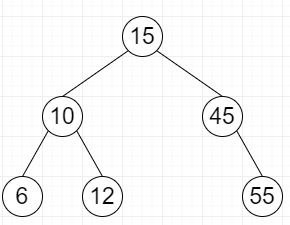# Construct a BST from given postorder traversal using Stack in Python

Suppose we have one postorder traversal of a binary search tree; we have to find the binary search tree from it.

So, if the input is like [6, 12, 10, 55, 45, 15], then the output willTo solve this, we will follow these steps −

• Define a function solve() . This will take postorder

• n := size of postorder

• root := make a new tree node with last element of postorder

• stk := a stack

• insert root into stk

• i := n - 2

• while i >= 0, do

• x := make a new node with value postorder[i]

• while stk is not empty and postorder[i] < value of top of the stk, do

• temp := top of stk

• delete top element from stk

• if temp is not null, then

• temp.left := x

• otherwise,

• right of the stk top element := x

• insert x into stk

• i := i - 1

• return root

• From the main method do the following −

• return solve(postorder)

## Example

Let us see the following implementation to get better understanding −

Live Demo

class TreeNode:
def __init__(self, data = 0):
self.val = data
self.left = None
self.right = None
def solve(postorder):
n = len(postorder)
root = TreeNode(postorder[n - 1])
stk = []
stk.append(root)
i = n - 2
while ( i >= 0):
x = TreeNode(postorder[i])
temp = None
while (len(stk) > 0 and postorder[i] < stk[-1].val) :
temp = stk[-1]
stk.pop()
if (temp != None):
temp.left = x
else:
stk[-1].right = x
stk.append(x)
i = i - 1
return root
def build_tree(postorder):
return solve(postorder)
def inord( node):
if node:
inord(node.left)
print( node.val, end = " ")
inord(node.right)
postorder = [6, 12, 10, 55, 45, 15]
root = build_tree(postorder)
print( "Inorder traversal:", end = " ")
inord(root)

## Input

[6, 12, 10, 55, 45, 15]

## Output

6 10 12 15 45 55